# Simulating fossils

#### 2022-04-19

This vignette provides information about how the package stores and outputs information about fossil sampling times via the fossils object and available options for simulating fossil recovery.

## Contents

### The fossils object

The fossils object contains information about fossil sampling times and their relationship to the corresponding phylo and taxonomy objects. In some simulation conditions we may know the age of fossils precisely. Alternatively, there may be some uncertainty associated with specimen ages, i.e. we only know the age to within some stratigraphic interval, which can be incorporated into the fossils object.

The object contains a dataframe with species and age information for each fossil with the following 4 fields:

• sp the label of the corresponding species. This label matches the edge labels in the corresponding phylo object or the species labels in the corresponding taxonomy object if taxonomic information was provided

• edge the label of the sampled node or tip in the phylogeny, i.e the node at the end of the edge along which the fossil was sampled

• hmin the age of the fossil or the youngest bound of the time interval in which the fossil was sampled

• hmax the oldest bound of the time interval in which the fossil was sampled. This is equal to hmin if exact sampling times are known

The object also contains a logical variable from.taxonomy indicating whether the fossil record was simulated using a taxonomy object, in which case from.taxonomy = TRUE, or from a phylo object in which case from.taxonomy = FALSE (the default).

## Constant fossil recovery

The simplest model of fossil recovery is a Poisson sampling process, where fossils are sampled along lineages with constant rate $$\psi$$ (function argument rate).

Fossils can be simulated using a phylo object or an existing taxonomy object generated using the function sim.taxonomy.

### Simulating fossils for a tree object

If a phylo rather than a taxonomy object is passed to the function, it will assume that all speciation has occurred via symmetric (i.e. bifurcating, $$\beta = 1$$) speciation, i.e. every edge in the tree represents a unique species. This applies to all functions used to simulate fossils.

# set the random number generator seed to generate the same results using the same code
set.seed(1234)

# simulate a tree using TreeSim conditioned on tip number
lambda = 1
mu = 0.2
tips = 8
t = TreeSim::sim.bd.taxa(n = tips, numbsim = 1, lambda = lambda, mu = mu)[]
# t is an object of class phylo
t
#>
#> Phylogenetic tree with 8 tips and 7 internal nodes.
#>
#> Tip labels:
#>   t8, t4, t3, t1, t6, t2, ...
#>
#> Rooted; includes branch lengths.

### The autocorrelated fossil recovery model

Under the autocorrelated model, traits evolve along lineages according to a Brownian motion process, where the strength of the relationship between ancestor and descendant values is determined by the parameter $$\nu$$ (function argument v). New trait values are drawn from a lognormal distribution, where the mean is equal to the value of the ancestor and the variance is a function of $$\nu$$ and the species or edge duration. If $$\nu$$ is small values will be more similar between ancestor and descendants, and if $$\nu$$ is zero all trait values will be equal. This is model is described in Heath et al. (2014) and is equivalent to the autocorrelated relaxed clock model described in Kishino et al. (2001).

# define the initial rate at the root or origin
rate = 1

# simulate rates under the autocorrelated trait values model (the default option)
rates = sim.trait.values(init = rate, tree = t, v = 0.01)
# simulated rates
rates
#>   0.9475371 0.8816897 0.8299168 0.8388761 0.8277377 0.8619949 0.7568962
#>   0.8853375 0.6971045 0.8407235 0.9940334 0.9321089 0.8348147 0.8843375
#>  0.8239667

# simulate fossils
f = sim.fossils.poisson(tree = t, rate = rates)

# plot the output
plot(f, tree = t)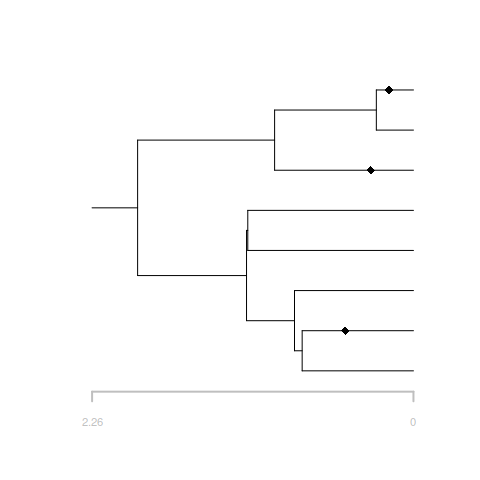### The independent fossil recovery model

Under the independent model a new trait value is drawn for each species from any valid user-specified distribution dist. To be valid the function just has to return a single value.

# define the initial rate at the root or origin
rate = 1

# define the distribution used to sample new rates
# in this case an exponential with mean = 1
dist = function() { rexp(1) }

# simulate trait values under the independent trait values model
rates = sim.trait.values(init = rate, tree = t, model = "independent", dist = dist)

# simulate fossils
f = sim.fossils.poisson(tree = t, rate = rates)

# plot the output
plot(f, tree = t)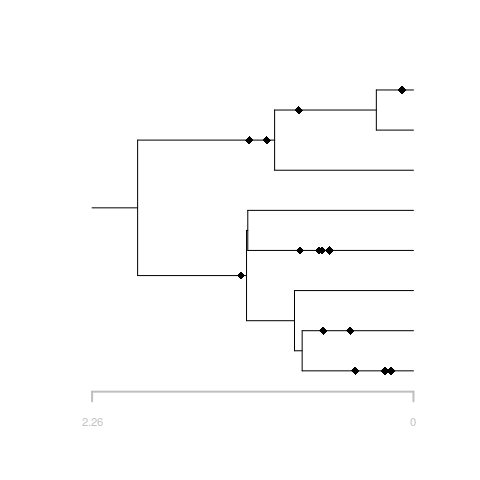The parameter change.pr is the probability that changes in trait values are coincident with speciation events. At each speciation event change occurs with probability change.pr and new values are drawn from any valid user-specified distribution dist. If change.pr = 1 a change will occur at every speciation event and the model will be the same as above.

# define the initial value at the root or origin
rate = 1

# define the distribution used to sample new rates
# in this case an exponential with a mean ~ 3
dist = function() { rexp(1, 1/4) }

# define the probability of the trait value changing at each speciation event
change.pr = 0.5

# simulate trait values under the independent trait values model
rates = sim.trait.values(init = rate, tree = t, model = "independent", dist = dist, change.pr = change.pr)

# simulate fossils
f = sim.fossils.poisson(tree = t, rate = rates)

# plot the output
plot(f, tree = t)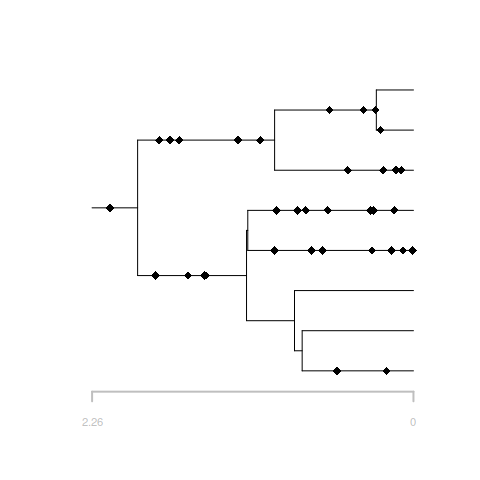### Environment and lineage-dependent fossil recovery

Lineage specific values generated using sim.trait.values can also be passed to the the function sim.fossils.environment to define the PD, DT, and PA parameters.

# define constant values for preferred depth and depth tolerance
PD = 2
DT = 0.5

# simulate lineage variable peak abundance values

# define the distribution used to sample new PA values
# in this case a uniform in the interval 0, 1
dist = function() { runif(1) }

# simulate trait values under the independent model
PA = sim.trait.values(init = 0.1, tree = t, model = "independent", dist = dist)

# simulate fossils
f = sim.fossils.environment(tree = t, interval.ages = times, proxy.data = wd, PD = PD, DT = DT, PA = PA)

# plot the output
plot(f, tree = t, show.strata = TRUE, interval.ages = times)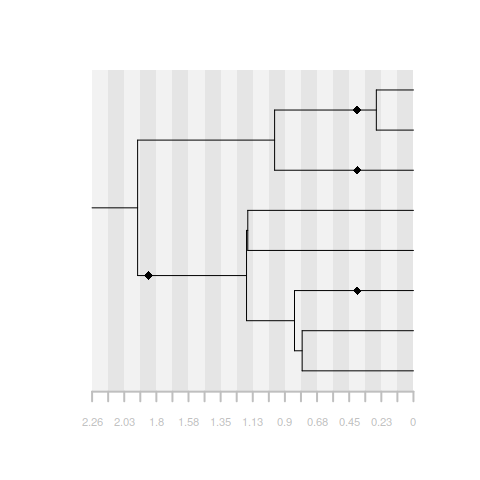## Extant species and tip sampling

The functions sim.extant.samples and sim.tip.samples can be used to simulate incomplete extant species and tip sampling, respectively. The parameter rho is the probability that an extant species or a tip will be sampled.

# simulate fossils
f = sim.fossils.poisson(tree = t, rate = rates)

# simulate extant species sampling
f2 = sim.extant.samples(fossils = f, tree = t, rho = 0.5)

# plot the output
plot(f2, tree = t, extant.col = "red")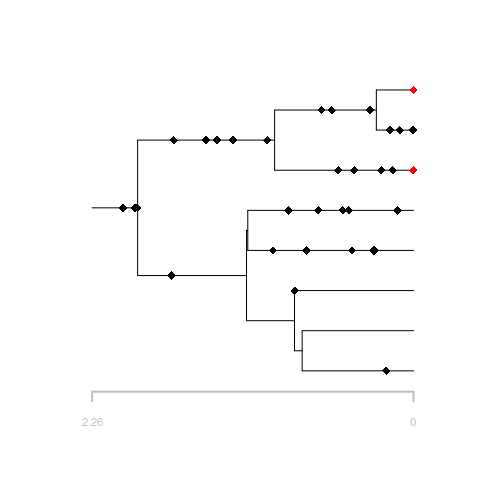# for tip sampling only, create a fossil object with no fossils
f = fossils()

# simulate tip sampling
f2 = sim.tip.samples(fossils = f, tree = t, rho = 0.75)

# plot the output
plot(f2, tree = t)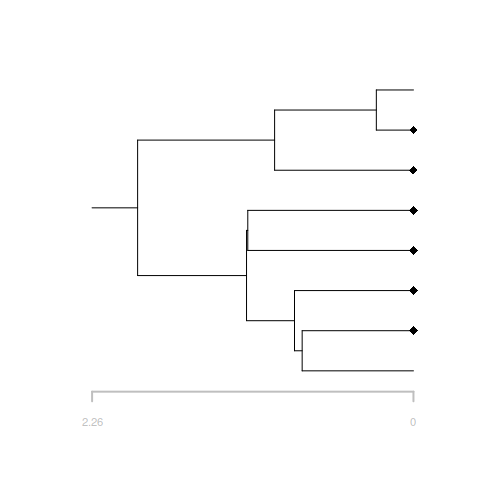See the paleotree vignette to see how FossilSim objects can be converted into paleotree objects.